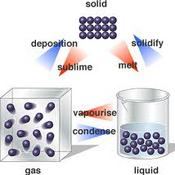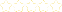• > carolyn fruin
• > A5 - Gases, Liquids and Solids
+# A5 - Gases, Liquids and Solids

Owner: carolyn fruin
##### Description:
Learning Targets: I can convert between pressure units (atm, mmHg, kPa). I can convert between Kelvin and degrees Celsius. I can explain that Kelvin temperature is always used in gas law calculations because 0 K actually means zero molecular motion whereas 0 degrees Celsius is simply the freezing point of pure water. I can identify a graph of two variables as directly proportional or inversely proportional. I can identify a what variables are given and unknown in gas law problems. I can explain what temperature and pressure look like in terms of the Kinetic Molecular Theory temperature = motion of particles (KE) pressure = collisions with the walls of the container I can solve stoichiometric problems using the molar volume of a gas at STP. I can recognize which value of R to use in an ideal gas law problem. I can use dimensional analysis solve for various variables in the ideal gas law as well as density and molar mass. I can explain that Graham’s Law explains why all gases act the same. I can state that heavy particles move slowly and lighter particles move faster. I can calculate how much faster the light particle is moving than the heavier particle.

### Your Quiz Points in this Playlist

Total Possible
0
5 Tutorials in this Playlist#### Unit 5 Syllabus

Author: carolyn fruin

#### Introduction to Gases - chapter 10

Author: carolyn fruin
Rating:(1)

#### Mathematics of Gases - chapter 11

Author: carolyn fruin
Rating:(2)

#### Solids, Liquids and Phase Changes (chapter 12)

Author: carolyn fruin
Rating:(21)

#### Gases WebQuest

Author: carolyn fruin
Rating:(13)